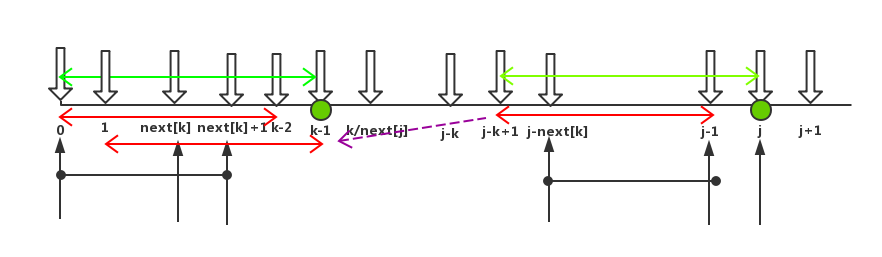# sofu6

 博客园  :: 首页  :: 新随笔  :: 联系 :: 订阅:: 管理

### 引言

KMP算法指的是字符串模式匹配算法，问题是：在主串T中找到第一次出现完整子串P时的起始位置。该算法是三位大牛：D.E.Knuth、J.H.Morris和V.R.Pratt同时发现的，以其名字首字母命名。在网上看了不少对KMP算法的解析，大多写的不甚明了。直到我看到一篇博客的介绍，看完基本了解脉络，本文主要是在其基础上，在自己较难理解的地方进行补充修改而成。该博客地址为：https://www.cnblogs.com/yjiyjige/p/3263858.html，对作者的明晰的解析表示感谢。

### 1. 一般的解法

KMP算法要解决的问题就是在字符串（也叫主串）中的模式（pattern）定位问题。说简单点就是我们平时常说的关键字搜索。模式串就是关键字（接下来称它为P），如果它在一个主串（接下来称为T）中出现，就返回它的具体位置，否则返回-1（常用手段）。A和E不相等，那就把i指针移回第1位（假设下标从0开始），j移动到模式串的第0位，然后又重新开始这个步骤：1 /**
2
3  * 暴力破解法
4
5  * @param ts 主串
6
7  * @param ps 模式串
8
9  * @return 如果找到，返回在主串中第一个字符出现的下标，否则为-1
10
11  */
12
13 public static int bf(String ts, String ps) {
14
15     char[] t = ts.toCharArray();
16
17     char[] p = ps.toCharArray();
18
19     int i = 0; // 主串的位置
20
21     int j = 0; // 模式串的位置
22
23     while (i < t.length && j < p.length) {
24
25        if (t[i] == p[j]) { // 当两个字符相同，就比较下一个
26
27            i++;
28
29            j++;
30
31        } else {
32
33            i = i - j + 1; // 一旦不匹配，i后退
34
35            j = 0; // j归0
36
37        }
38
39     }
40
41     if (j == p.length) {
42
43        return i - j;
44
45     } else {
46
47        return -1;
48
49     }
50
51 }

### 2.如果人眼来优化的话，怎样处理P[0 ~ k-1] == P[j-k ~ j-1]上面说的,如果分解成计算机的步骤，则是如下的过程：

1）找出前缀pre，设为pre[0~m]；

2）找出后缀post，设为post[0~n]；

3）从前缀pre里，先以最大长度的s[0~m]为子串，即设k初始值为m,跟post[n-m+1～n]进行比较：

如果相同，则pre[0~m]则为最大重复子串，长度为m，则k=m;

如果不相同，则k=k-1;缩小前缀的子串一个字符，在跟后缀的子串按照尾巴对齐，进行比较，是否相同。

如此下去，直到找到重复子串，或者k没找到。

### 3.求next数组

 1 public static int[] getNext(String ps) {
2
3     char[] p = ps.toCharArray();
4
5     int[] next = new int[p.length];
6
7     next = -1;
8
9     int j = 0;
10
11     int k = -1;
12
13     while (j < p.length - 1) {
14
15        if (k == -1 || p[j] == p[k]) {
16
17            next[++j] = ++k;
18
19        } else {
20
21            k = next[k];
22
23        }
24
25     }
26
27     return next;
28
29 }1 public static int KMP(String ts, String ps) {
2
3     char[] t = ts.toCharArray();
4
5     char[] p = ps.toCharArray();
6
7     int i = 0; // 主串的位置
8
9     int j = 0; // 模式串的位置
10
11     int[] next = getNext(ps);
12
13     while (i < t.length && j < p.length) {
14
15        if (j == -1 || t[i] == p[j]) { // 当j为-1时，要移动的是i，当然j也要归0
16
17            i++;
18
19            j++;
20
21        } else {
22
23            // i不需要回溯了
24
25            // i = i - j + 1;
26
27            j = next[j]; // j回到指定位置
28
29        }
30
31     }
32
33     if (j == p.length) {
34
35        return i - j;
36
37     } else {
38
39        return -1;
40
41     }
42
43 }

### 4.next数组求解算法优化public static int[] getNext(String ps) {

char[] p = ps.toCharArray();

int[] next = new int[p.length];

next = -1;

int j = 0;

int k = -1;

while (j < p.length - 1) {

if (k == -1 || p[j] == p[k]) {

if (p[++j] == p[++k]) { // 当两个字符相等时要跳过

next[j] = next[k];

} else {

next[j] = k;

}

} else {

k = next[k];

}

}

return next;

}

posted on 2018-07-24 22:54  sofu6  阅读(118854)  评论(27编辑  收藏  举报